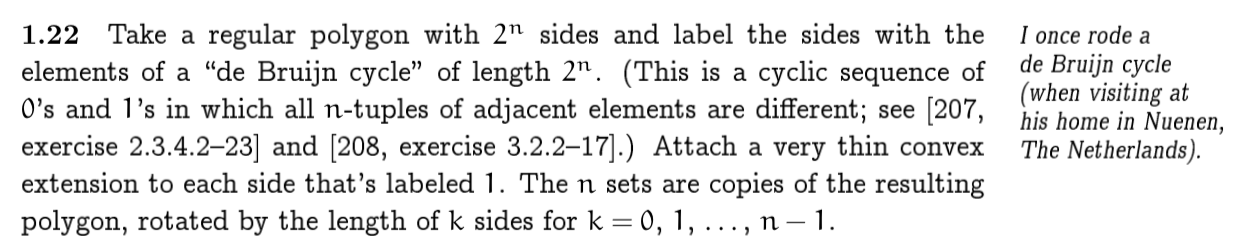# A brief tour of Digital Assets

## Why Protocol/Standard is imporatnt

• Infrastructure
Bitcoin/Ethereum/Cosmos/…
• Protocol / Standard
ERC20/ERC721/Bancor/Uniswap
• Application
Cryptokitties/Unisock

## What is EIP

• 草案(Draft)
• 活躍(Active)
• 最後召集(Last Call)
• 最終(Final)
• 被棄用(Abandoned)
• 被取代(Superseded)

• 標準提案 A Standard Track EIP
• 核心提案 Core
• 網路提案 Networking
• 介面提案 Interface
• 應用標準提案 ERC(Ethereum Request for Comments)
• 元提案 meta-EIP(Process EIP)
• 信息提案 Informational EIP

## A Briefly Tour of Token Standard

ERC tl;dr
ERC20 ICO
ERC223 防黑洞
ERC621 可增減總量
ERC827 可授權代幣
ERC875 Alpha Wallet 用來賣門票的（Simple Atomic Swaps）
ERC1726 可分潤代幣（P3D）
ERC721 加密貓/收藏品
ERC777 將授權轉賬從兩步鏈上操作變為一步
ERC1155 Enjin 用來做遊戲道具的

## ERC777

ERC777 是出現在 OpenZepplin 合約倉庫 里的，除了 ERC20 和 ERC721 之外的第三個 Token 標準，其重要性和適用性不言而喻。
（TBD）

（TBD）

## How to Migrate High Quality Assets into Crypto

Blockchains collapse the cost of instrumentation, issuance and exchange at zero marginal cost!
—— WTF is Capital: How to Fix Capitalism

Blockchains collapse the cost of instrumentation，這也是整個 Crypto 領域最有價值的地方。無論是 UMA、Market Protocol 還是 Synthetix 大家似乎都是想 Tokenize Everything，但是事實上 at this moment，並不是所有的事情都適合去代幣化。適合被遷移的資產要考慮以下三個方面：

• 價值
• 摩擦
• 流動性

### 房地產

every lawyer whose career needs a boost is now a 「crypto lawyer」 and they』re making bank even as the tech and culture languish into utter inanity
—— Size Does Matter

—— Incubator

（TBD）

（TBD）

（TBD）

（TBD）

### 數據

I am selling some of my time & secrets on @gitcoin
10 Dai / Month，All my draft tweets, raw design files & private meetups.
30 Dai / Month，The secrets, rumors and leaks I get. My trades & ideas.
—— @ricburton

（TBD）

# Divisor function

《數學與猜想》第一卷里第二精彩的段落，就是關於歐拉發現除數函數的性質的部分了，Polya 直接用了完整的一章來轉載 這篇研究報告

## 參考資料

http://www.kdsrpg.com:10099/svn/canvasbuilder

# 巴塞爾問題

$$\sum_{n=1}^\infty \frac{1}{n^2} = \frac{\pi^2}{6}$$

《數學與猜想》是 How to solve it 的續集，相比《怎樣解題》這本小冊子，《數學與猜想》提供了更多的材料。其中令我印象最為深刻的，就是 巴塞爾問題 了。參見《數學與猜想》第一卷、第二章、第六節 —— 由類比作出的發現。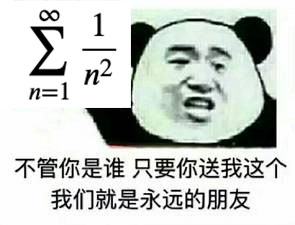## 由類比作出的發現 —— 歐拉最初的推導

$$\sin x = x – \frac{x^3}{3!} + \frac{x^5}{5!} – \frac{x^7}{7!} + \cdots$$

$$\frac{\sin x}{x} = 1 – \frac{x^2}{3!} + \frac{x^4}{5!} – \frac{x^6}{7!} + \cdots$$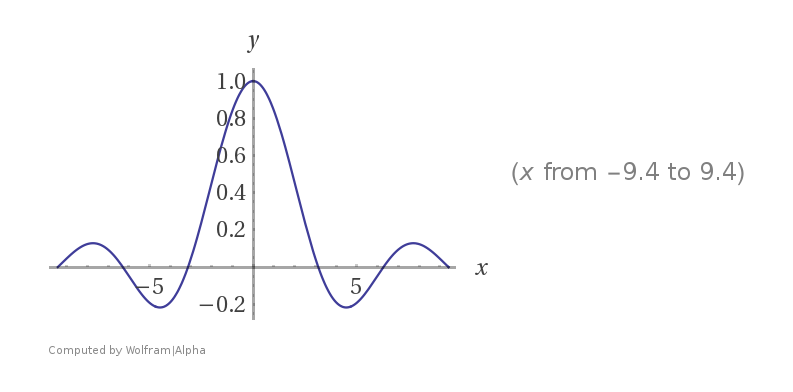\begin{align} \frac{\sin x}{x} &= \left(1 – \frac{x}{\pi}\right)\left(1 + \frac{x}{\pi}\right)\left(1 – \frac{x}{2\pi}\right)\left(1 + \frac{x}{2\pi}\right)\left(1 – \frac{x}{3\pi}\right)\left(1 + \frac{x}{3\pi}\right) \cdots \newline \ &= \left(1 – \frac{x^2}{\pi^2}\right)\left(1 – \frac{x^2}{4\pi^2}\right)\left(1 – \frac{x^2}{9\pi^2}\right) \cdots \end{align}

$$\frac{1}{3!} = \frac{1}{6} = -\left(\frac{1}{\pi^2} + \frac{1}{4\pi^2} + \frac{1}{9\pi^2} + \cdots \right) = -\frac{1}{\pi^2}\sum_{n=1}^{\infty}\frac{1}{n^2}.$$

$$\sum_{n=1}^\infty \frac{1}{n^2} = \frac{\pi^2}{6}$$

## 黎曼 Zeta 函數

$$\zeta(s) = \sum_{n=1}^\infty \frac{1}{n^s}$$

$$\zeta(2n) = \frac{(2\pi)^{2n}(-1)^{n+1}B_{2n}}{2\cdot(2n)!}$$# On Venn Diagram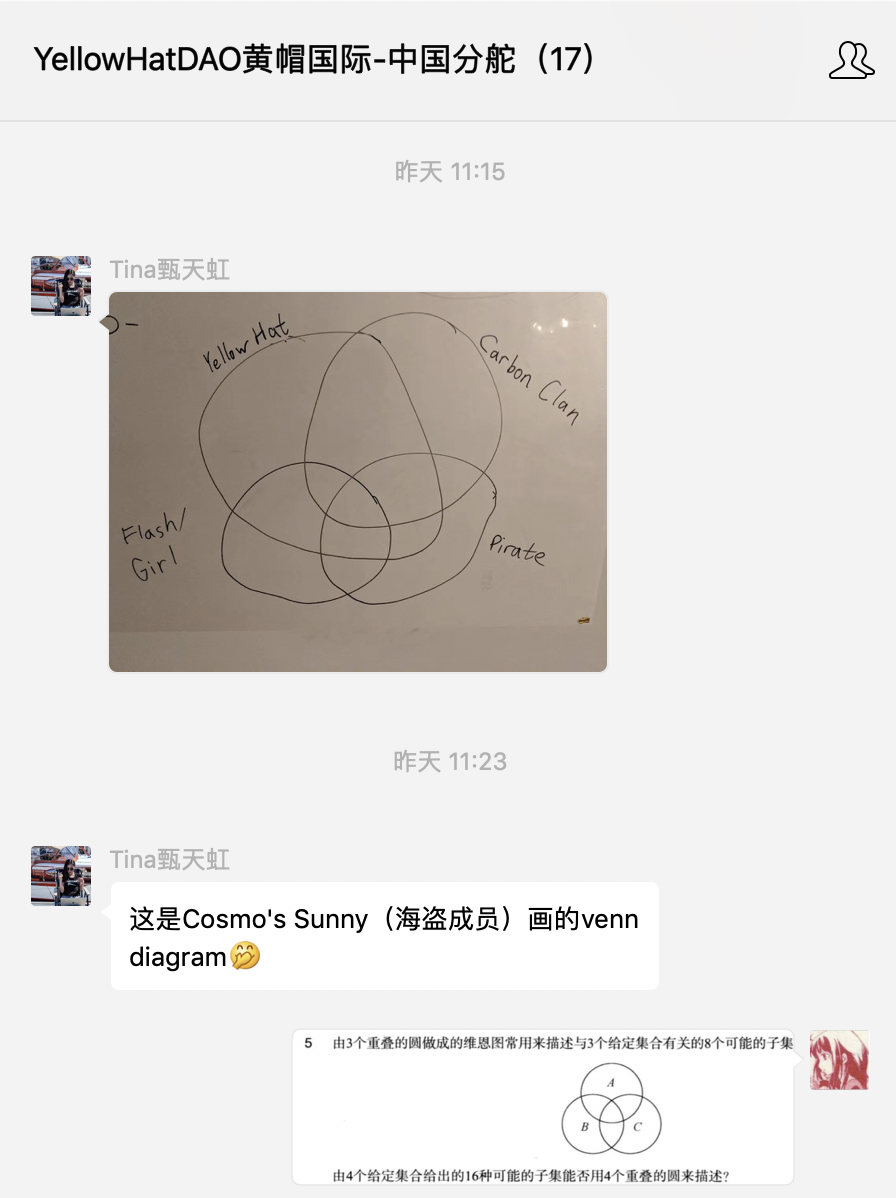。。仔細看的畫會發現少了一個區域。。。正好這幾天在和 紙喵 一起看《混凝土數學》，所以立刻就去吐槽了。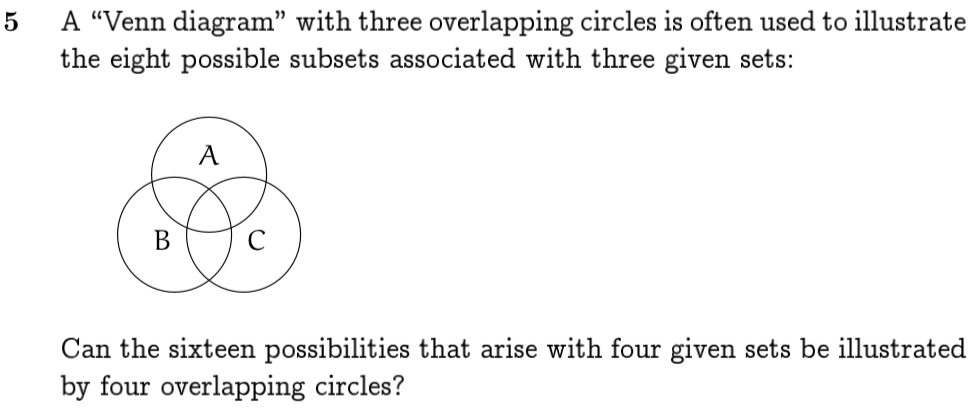David 很快還給出了一個 5 階的圖。。。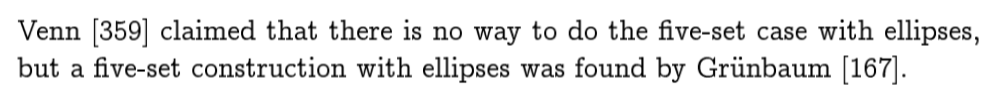Show that it’s possible to construct a Venn diagram for all 2^n possible subsets of n given sets, using n convex polygons that are congruent to each other and rotated about a common center.